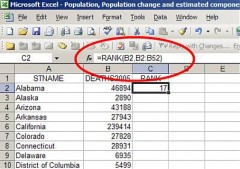# What formula would you use to calculate the average of your monthly expenses in Excel?The quick and simple answer to this question would be to calculate how much your total expenses amount to for the month are and divide it by the total number of individual expenses you have, giving you the average value of each expense over the whole month. Excel will calculate this for you, the formula for which would be =average([Cell1]:[Cell2])

But, I suspect this is not exactly the answer you are looking for ...

There are many free tools available on the internet for recording and managing your budget each month and most of them are Excel spreadsheet-based, enabling you to easily input monthly expenditures, leaving the spreadsheet to calculate the bottom line for you.

Having completed a review of the many tools available, financial expert, Dave Ramsey’s range of free budgeting spreadsheets details a comprehensive log of monthly expenditure in an easy to use format.  Visit his site and download the range of free tools.  www.daveramsey.com/tools/budget-forms/

thanked the writer.
Just add up the total expenses of the month, and divide by the number of days in the month.
thanked the writer.